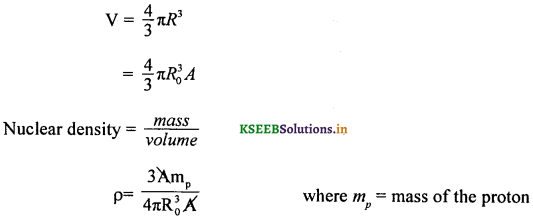# 2nd PUC Physics Question Bank Chapter 13 Nuclei

Students can Download 2nd PUC Physics Chapter 13 Nuclei Questions and Answers, Notes Pdf, 2nd PUC Physics Question Bank with Answers helps you to revise the complete Karnataka State Board Syllabus and to clear all their doubts, score well in final exams.

## Karnataka 2nd PUC Physics Question Bank Chapter 13 Nuclei

### 2nd PUC Physics Nuclei One Marks Questions and Answers

Question 1.
Mention Einstein’s mass energy relation.
E = me2 where E is the energy equivalent of a mass m and c is the velocity of light in vacuum.

Question 2.
Give the relation between amu and MeV,
1 amu = 931 MeV.

Question 3.
What is the relation between the radius of a nucleus and mass number of the corresponding element?
R = R0A1/3 where R is the radius of the nucleus and R0 is called fermi radius whose value ranges from 1.2 fm to 1.4 fm.Question 4.
What is meant by specific binding energy?
Binding energy per nucleon is called specific binding energy.

Question 5.
What is a nuclear reactor?
A nuclear reactor is a device in which self sustained controlled nuclear chain reaction takes place.

Question 6.
What is meant by mass number?
The total number of protons and neutrons in a nucleus is called its mass number.

Question 7.
Do nuclear forces obey the inverse square law?
Nuclear forces do not obey the inverse square law.

Question 8.
What is mass defect?
Mass defect is the difference between the sum of the masses of the nucleons forming the nucleus and its rest mass. ∆m = [Zmp + Zme + (A – Z)mn]-matom

Question 9.
On what factors docs the stability of a nucleus depend?
The stability of a nucleus depends on the relative number of protons and neutrons in the nucleus.

Question 10.
What is binding energy?
Energy equivalent of mass defect is called Binding energy. BE = (∆m)c2 = (∆m in a.m.u) (931) MeV.

Question 11.
Why are nuclear forces called exchange forces?
This is because the force between two nucleons is due to the exchange of π-mesons between them.

Question 12.
What is the nature of nuclear force?
Nuclear force is strongly attractive in nature.Question 13.
What are Nuclear forces?
Nuclear forces bind the nucleons together.

Question 14.
What is the range of nuclear forces?
The range of nuclear forces is upto 10-15 m.

Question 15.
Mention the order of nuclear density.
The order of nuclear density is 10-17 kg m-3 irrespective of the size of the nucleus.

Question 16.
What is meant by nuclear fission?
The process by which a heavy nucleus splits into two or more lighter nuclei of comparable masses is known as nuclear fission.

Question 17.
What is the function of moderator in a nuclear reactor?
A moderator slows down neutrons to thermal energy levels.

Question 18.
What is nuclear fusion?
The process by which two light nuclei combine to form a heavier nucleus with liberation of energy is called nuclear fusion.

Question 19.
Define critical size.
A fissionable material of a particular size for which a chain reaction takes place at a constant rate is called its critical size.

Question 20.
What is the magnitude of the charge on a neutron?
Magnitude of the charge on a neutron is zero.

Question 21.
How many kg of mass of a substance make 1 amu?
1 amu = 1.66054 × 10-27kg.

Question 22.
What is the function of a breeder reactor?
A breeder reactor converts fertile material into fissile material. In the case of a nuclear power reactor, nuclear energy is converted into electrical energy.Question 23.
What is the function of cadmium rods in a nuclear reactor?
Cadmium rods absorb neutrons and hence control the rate of reaction.Question 24.
What are the two types of reactors?
Research reactors and breeder reactors.

Question 25.

Question 26.

Question 27.
What is an α particle?
An α-particle is a helium nucleus consisting of two protons and two neutrons.

Question 28.
What are β particles?
β-particles are electrons emitted by the nucleus when neutrons decay.

Question 29.
γ-rays are electromagnetic radiations emitted while an excited nucleus returns to the ground state.

Question 30.
A radioactive sample is placed in boiling water. Will its activity increase?
No. Its activity will not vary with temperature.

Question 31.
Can a powerful magnetic or electric field deflect γ rays?
No. γ – rays cannot be deflected by electric or magnetic fields,

Question 32.
What is the velocity of γ radiations?
γ – radiations travel with the velocity of light which is 3 × 108 ms-1.

Question 33.
Can a radioactive sample emit all types of radiations at the same time?
At a given instant a radioactive sample can emit only one type of radiation.

Question 34.
What is the charge of an α particle?
Charge on an α particle is + 2e.

Question 35.
α-rays undergo scattering.Question 36.
State the law of radioactive disintegration. (March 2014)
The rate of disintegration of a radioactive sample at any instant is directly proportional to the number of nuclei present in the sample at that instant.

Question 37.
Define decay constant.
Decay constant is defined as the reciprocal of the time during which the number of nuclei of the sample reduces to $$\frac { 1 }{ e }$$ of its initial value.

Question 38.
What is a positron?
Positron is an anti particle of electron. Its mass is equal to that of electron. Its charge is equal in magnitude but opposite in sign.

Question 39.
What is mean life of a radioactive element?
Mean life of a radioactive sample is the ratio of the total life times of all the individual nuclei to the total number of nuclei present in the sample.

Question 40.
Define curie.
The activity of substance is one curie if the rate of disintegration of nuclei present in the sample is 3.7 × 1010 per second.

Question 41.
The phenomenon by which lighter elements are made artificially radioactive by the process of transmutation is called artificial radioactivity.

Question 42.

Question 43.
Mention the isotope used for dating ancient samples.
Radio carbon-14 is used for dating ancient samples.

Question 44.
Name any one isotope used in the feild of medicine.
Radioiodine is used in curing overactive thyroid glands.

Question 45.
Write the expression for the half life of a radioactive element.
T1/2 = $$\frac{0.693}{\lambda}$$ where T1/2 → half life and λ is the decay constant.Question 46.
What is the SI unit of activity?
Becquerel is the SI unit of radioactivity.

Question 47.
What is the relation between curie and becquerel?
1 Ci = 3. × 1010 Bq (1 Bq = 1 disintegration per second)

Question 48.
Define half life of a radioactive element.
Half life of a radioactive sample of an element is defined as the time taken for half the number of nuclei in the sample to disintegrate.

Question 49.
Write the relation between mean life and decay constant.
τ = $$\frac{1}{\lambda}$$ where t → mean life, λ → decay constant.

Question 50.
What is meant by activity of a sample?
The time rate of disintegration of atoms is called the activity of a sample.

### 2nd PUC Physics Nuclei Two Marks Questions and Answers

Question 1.
What are atomic and mass numbers?
Atomic number is the number of protons in the nucleus which is represented by Z. The total number of nucleons (protons + neutrons) inside a nucleus is called mass number which is represented by A.

Question 2.
What is nuclear mass? How is it calculated?
Nuclear mass is the mass of all the nucleons present inside the nucleus and is calculated by M = ZMp + (A – Z)Mn where Mp and Mn are masses of protons and neutrons respectively.

Question 3.
Calculate the energy equivalent of 1 kg of mass.
Energy equivalent of 1 kg of mass = 1 × (3 × 108)2 = 9 × 1016 J.

Question 4.
Explain nuclear chain reaction.
When the nucleus of U238 undergoes fission, 2 or 3 neutrons are released with fission fragments and energy. These neutrons dan cause fission in other nuclei producing more neutrons. The process continues till all the nuclei of the sample undergo nuclear fission. This is called nuclear chain reaction.

Question 5.
Explain nuclear binding energy.
Nuclear binding energy is defined as the energy required to break up a nucleus into its constituent nucleons. It is numerically equal to the energy equivalent of mass defect.
∴ Binding energy = Δm × c2 = Δm × 931 MeV.

Question 6.
Give an example for exothermic and endothermic processes.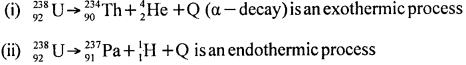For exothermic processes, Q is +ve and for an endothermic process, ‘Q’ is -ve.Question 7.
Outline the method of converting non – fissionable Uranium – 238 into fissionable Plutonium – 239.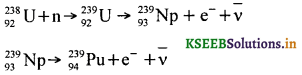where 23994Pu undergoes fission with slow neutrons.

Question 8.
Why do nuclear fusion reactions take place at a very high temperature?.
The nature of force between two protons is repulsive. To overcome the strong repulsive force between them, these are raised to a very high temperature. By doing so, the protons fuse with each other forming a heavier nucleus.

Question 9.
What is an isotope? Give an example.
An atom whose atomic mass number is different but the atomic number is the same as that of another atom of the same element is called an isotope.
e.g: Isotopes of 1H1 are 1H2 and 1H3.

Question 10.
What is an isobar? Give an example.
The element having the same atomic mass number but a different atomic number to that of another element is called an isobar.
e.g: 1H3 and 2He3 are isobars.

Question 11.
What is an isotone? Give an example.
The atom of an element having the same number of neutrons as that of the atom of another element is called an isotone.
e.g: 2He4 and 3Li5 are isotones.Question 12.
Draw the block diagram of a nuclear reactor.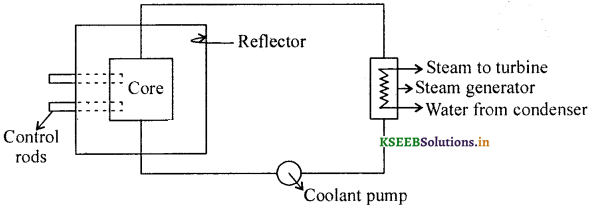Question 13.
Mention any two methods of disposal of nuclear waste.
Methods of disposal of nuclear wastes:

1. Nuclear waste taken in lead containers are buried under salt mines/deposit.
2. Nuclear waste is converted into silicates and then buried under rocks.

Question 14.
Draw a neat labelled specific binding energy curve.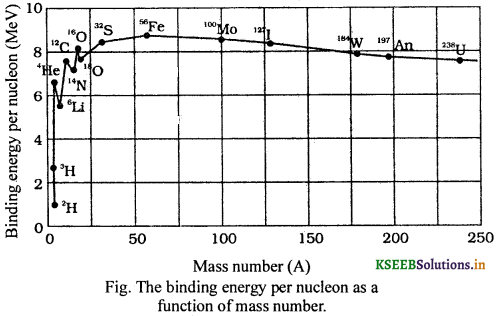### 2nd PUC Physics Nuclei Three Marks Questions and Answers

Question 1.
Show that 1 amu = 932 MeV.
From Einstein’s relation E = mc2.
m = 1 amu = 1.661 × 10-27 kg and c = 2.998 108 ms-1
E = 1.661 × 10-27 × (2.998 × 108)2 = 14.929 × 10-11 J
= $$\frac{14.929 \times 10^{-11}}{1.602 \times 10^{-19}}$$ ev = 9.318 × 108 MeV = 931.8 MeV
∴ Energy equivalent of 1 amu = 932 MeV.

Question 2.
Define amu and eV. Mention the relation between them.
Atomic mass unit (amu) is $$\frac { 1 }{ 12 }$$ of the mass of a carbon atom in its lowest energy state. One electron volt (e V) is the energy gained by an electron when it is accelerated through a potential difference of 1 V.
1 amu = 931.8 MeV.Question 3.
Name the different types of nuclear reactors.
Nuclear reactors are classified into three categories:

• Research reactors
• Breeder reactors
• Power reactors.

Question 4.
Explain the nuclear fusion reaction with an example.
A type of reaction in which two or more lighter nuclei under a very high pressure and 106 K of temperature, combine to form a heavier nucleus with the release of enormous energy per nucleon is called nuclear fusion reaction.Energy released per nucleon is approximately 27 MeV/fusion.

Question 5.
Distinguish between a controlled and an uncontrolled chain reaction.
In a controlled chain reaction the neutron population is allowed to reach a steady state and thereafter the number of fission producing neutrons is maintained constant. This principle is used in the power reactor.

In an uncontrolled chain reaction the number of neutrons is allowed to multiply indefinitely in a very short interval. The entire mass undergoes fission and huge quantity of energy is released. This causes violent explosion.

Question 6.
The radiations emitted by radioactive elements are called radioactive radiations. The phenomenon of spontaneous disintegration of certain unstable nuclei of higher atomic mass number with the emission of α, β and γ radiations is called natural radioactivity. Radioactivity produced artificially or induced by transmutation is called artificial or induced radioactivity.

Question 7.
Give any three properties of α-rays.

1. α rays are deflected by electric and magnetic fields.
2. They affect photographic plates.
3. Their ionizing power is 100 times that of β-rays.Question 8.
Give any three properties of β-rays.

1. β-rays are deflected by electric and magnetic fields.
2. They affect photographic plates.
3. Their ionising power is $$\left(\frac{1}{100}\right)$$ that of α-particles.

Question 9.
Give any three properties of γ- radiations.

1. γ-rays are not deflected by electric and magnetic fields.
2. They travel with the velocity of light.
3. They affect photographic plates.

Question 10.
Mention any three applications of radio isotopes.

1. Radio phosphorus is used in agriculture to determine the kind of phosphate required for a given soil and crop.
2. Radio-iodine is used in the treatment of over active thyroid glands.
3. Radio-cobalt is used in the treatment of cancer.
Other applications:
4. Radio-carbon is used to estimate the age of fossils and archaeological specimens.
5. Radio-cobalt is used to detect flaws in the rubber tyres and metal castings.

Question 11.
Give an example for β decay followed by γ – decay (gamma decay).γ – photons of energy 1.17 MeV and 1.33 MeV are radiated as a result of daughter element returning to the normal state.Question 12.
Name the particle discovered in the process of β+ and β decay with an example.
(i) When a neutron decays to proton, an antineutrino particle is released.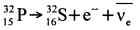(ii) When a proton decays to neutron, a neutrino particle is released.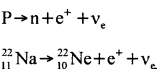Question 13.
What will be the position of the daughter nucleus in the periodic table, when β+ and β decays take place? Given an example under each.
When an element undergoes β decay, the daughter element shifts one group to the right. When an element undergoes β+ decay, the daughter element shifts one group to the left.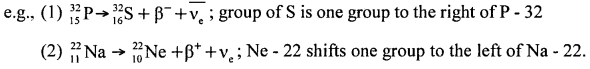Question 14.
Define and explain K – factor (multiplication factor) with respect to a nuclear fission chain reaction.
K = $$\frac{\mathrm{N}_{\mathrm{p}}}{\mathrm{N}_{\mathrm{A}}+\mathrm{N}_{\mathrm{L}}}$$
Where,
Np = Number of neutrons produced
NA = Number of neutrons absorbed
NL = Number of neutrons lost.
If K = 1, then the chain reaction becomes critical or self sustained.
If K > 1 then the chain reaction becomes uncontrollable. The reactor becomes super critical and explode.
If K < 1 then the chain reaction will not be self – sustained.Question 15.
Write the nuclear reaction equations for
(i) α – decay of 22688Ra
(ii) β – decay of 21083Bi
(iii) Electron capture of 12054Xe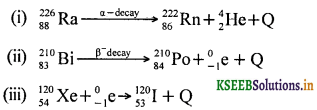Question 16.
Define the average time period of a radio active element.
The average time period of a radio active element is defined as the ratio of sum of lives of all the atoms to the total number of atoms present in the radio active sample.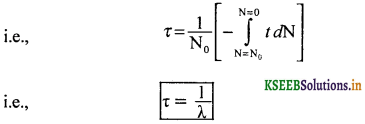Question 17.
Outline any three main features of specific binding energy curve.
The main features of specific binding energy curve :

1. The binding energy per nucleon is practically constant for nuclei of mass number of atoms (30 < A < 170).
2. The curve has a maximum specific binding energy of about 8.75 MeV for A = 56 and has value of 7.6 MeV for A = 238.
3. The specific binding energy is lower for both light nuclei (A < 30) and heavy nuclei (A > 170).

Question 18.
Write any three conclusions drawn from the features of specific binding energy curve.
The conclusions drawn from the features of specific binding energy curve:

1. The nuclear force is strong and attractive and produce binding energy of a few MeV per nucleon.
2. The constant specific binding energy for 30 < A < 170 implies that the nuclear force is a short range and saturated force.
3. For nuclei A ≥ 240, nuclei can be broken into smaller nuclei by bombarding them with fast moving projectiles.
4. For light nuclui (A ≤ 10), the specific binding energy of the fused heavier nuclei is more than the lighter nuclei.### 2nd PUC Physics Nuclei Five Marks Questions and Answers

Question 1.
What are the characteristics of nuclear forces? (July 2014)
Nuclear forces are,

1. strongly attractive.
2. short range forces.
3. charge independent.
4. non-gravitational.
5. exchange forces.
6. non-central forces.
7. saturated forces.

Question 2.
Write a note on nuclear fission chain reaction.
Nuclear fission is the phenomenon in which a heavy nucleus breaks up into lighter nuclei of nearly equal masses with liberation of energy
e.g.: When 92U235 is bombarded by a neutron, an isotope of uranium is formed which further breaks up into Xenon and Strontium.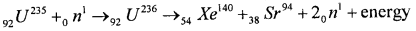In the above relation a mass defect of 0.216 amu occurs. This is equivalent to energy released of the order 200 MeV.
The products of fission are not unique. 92U235 may also undergo fission splitting into 56Ba141 and 36Kr92. The reaction is represented by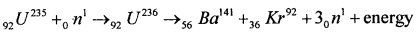The energy released usually appears in the form of K.E. of fission fragments and neutrons. Some of the energy is also released in the form of γ rays, heat energy and light energy. The fission, temperature and pressure become very high. Further, the fission fragments are usually radioactive and they become stable by emitting α rays and β rays.Question 3.
Explain the nuclear fusion reaction with an example.
Nuclear fusion is a process in which two lighter nuclei combine to form a single heavy nucleus releasing an enormous amount of energy. The reaction takes place at one. million kelvin of temperature and thousands of atmospheric pressure.

e.g: proton-proton cycle: The initial reaction of the proton-proton cycle is the formation of deuteron by the direct combination of two protons accompanied by the emission of a positron.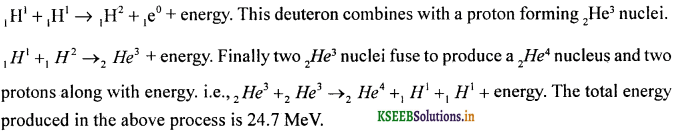It is estimated that the sun has been radiating energy at the rate of 3.8 × 1016 J/s for several billion years. It is known from the study of sun and the other stars that the most abundant nucleus in the stars is hydrogen.

Question 4.
Write a note on nuclear binding energy.
Binding energy of a nucleus is defined as the energy required to break up a nucleus into its constituent nucleons. It is numerically equal to the energy, equivalent to mass defect called binding energy.
∴ Binding energy = Δm × c2 = Δm × 931 MeV
The binding energy per nucleon is called specific binding energy. This quantity is the measure of stability of the nucleus. Larger the value of specific binding energy greater is the stability for the nucleus.

A graph of binding energy per nucleon versus mass number is the binding energy curve. The binding energy per nucleon has a low value for both very light and heavy nuclei. In order to attain a higher value of binding energy per nucleon, lighter nuclei must unite together to form a heavier nucleus leading to nuclear fusion.Question 5.
Distinguish between nuclear fission and nuclear fusion.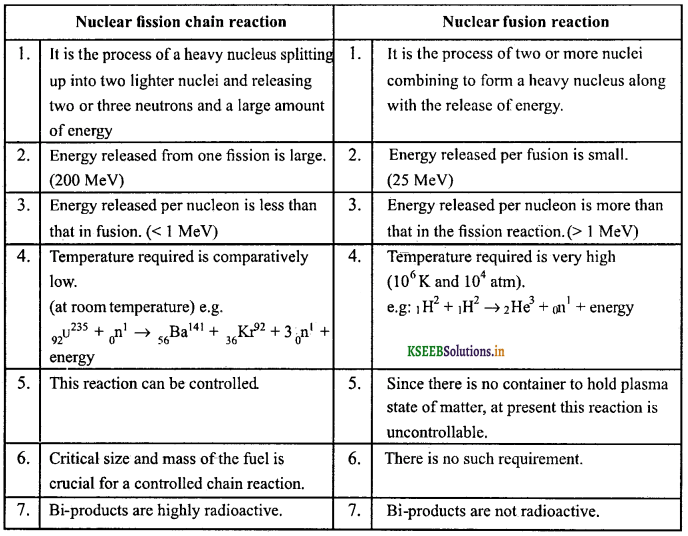Question 6.
State the law of radioactivity and hence, show that N = N0 e-λt.
Radio active law: The rate of disintegration of radio active atoms present. in the sample of an element is directly proportional to the number of radioactive atoms present at that instant.
i.e., $$\frac{d N}{d t}$$ ∝ N
or $$\frac{d v}{d t}$$ = -λN where ‘λ’ is known as the disintegration constant. The -ve sign indicates that the number of radio active nuclei/atoms decreases with the passage of time.
Hence $$\frac{d N}{N}$$ = -λ dt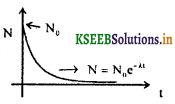Integrating both sides we get
loge = -λt + C
Applying the initial condition for t = 0, N = N0
We get, C = loge N0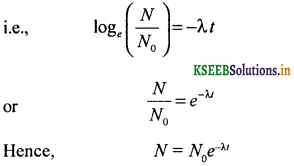A graph ‘N’ v/s time ‘t’ gives the exponential curve as shown in the figure.

Question 7.
Define ‘half life’, ‘decay constant’ and ‘mean life’ of a radioactive element and write the relation connecting them. (July 2014)
1. Half life of a radioactive sample is the time taken for half the number of nuclei to disintegrate. It is denoted by T1/2.

2. Mean life of a radioactive sample is defined as the ratio of sum of life times of all the individual nuclei to the total number of nuclei represent in the sample. It is denoted by τ.

3. Decay constant of a radioactive substance is defined as the reciprocal of the time during which the number of nuclei of the sample reduces to $$\frac{1}{e}$$ or 37% of its initial value.

4. Relation connecting half life, mean life and decay constant:
Half life T1/2 = $$\frac{0.693}{\lambda}$$ ….. (1)
Mean life τ = $$\frac{1}{\lambda}$$ ….. (2)
Dividing (1) by (2) then we have
$$\frac{T_{1 / 2}}{\tau}$$ = 0.693
∴ T1/2 = 0.693τ.Question 8.
Define half life of a radioactive element and deduce the expression for the same.
Half life of a radioactive sample is the time taken for half the number of nuclei to disintegrate. It is denoted by $$T_{\frac{1}{2}}$$. From the decay equation Nt = N0e-λt, it follows that for t → ∞, Nt → 0.
Thus it takes an infinitely long time for all the nuclei of any radioactive sample to disintegrate. But the time taken for half the number of nuclei in a given sample to disintegrate is finite and is characteristic of the given sample. It is called the half life of the radioactive substance.Thus half life of a radioactive substance is inversely proportional to its decay constant.

Question 9.
Give a brief account on the application of radio isotopes.
(a) Radio isotopes are used to eliminate harmful pests which destroy crops.
(b) Radio carbon (C14) is used to study the kinetics of plants photosynthesis.
(c) Radio isotopes are used to manufacture self luminous paints.
(d) Metallurgists use radio isotopes in the study of alloys.
(e) Radiation from radio isotopes is used for uniform mixing of lubricants and greases.
(f) Radio phosphorous is used in treating skin diseases.
(h) Radio isotopes are used in estimating the age of the earth.

Question 10.
Explain the parts of a nuclear power reactor.
The main parts of a nuclear reactor are
(a) Nuclear fuel
(b) Moderator
(c) Control rods
(d) Nuclear reflector
(e) Cooling system
(f) Safety system.

(a) Nuclear fuel: The fissionable material used in the reactor is called nuclear fuel. It may be natural uranium which contains only 0.02% of 92U235.

(b) Moderator: Moderator is used to slow down the neutrons. Commonly used materials are heavy water and graphite.

(c) Control rods: Control rods are used to control the rate of reaction by absorbing neutrons, e.g.: Boron steel, Cadmium steel, Silver along with Cadmium (alloy).

(d) Neutron reflector: Neutron reflectors are used to avoid leakage of neutrons from the surface.

(e) Coolant: The material used to extract the heat generated as a chain reaction is called coolant, e.g.: Ordinary water, heavy water, air, Carbon dioxide, molten sodium.

(f) Shield: To protect the surroundings from harmful radiations the reactor is enclosed within walls called protective.shield.

(e) Working: Neutrons produced by the action of α-particles on polonium cause fission of 92U235 This releases 200 MeV. Cadmium rods absorb neutron. Moderator slow down the neutron. The heat released is cooled by coolant. The steam is, used to run the turbine and generates electricity.### 2nd PUC Physics Nuclei List of Formulae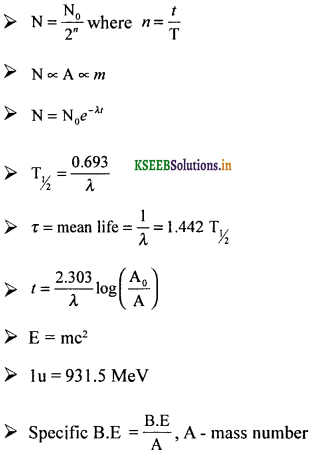### 2nd PUC Physics Nuclei Numericals and Solutions

Question 1.
A given coin has a mass of 3.0g. Calculate the nuclear energy that would be required to separate all the neutrons and protons from each other. F0r simplicity, assume that the coin is entirely made of 6329Cu atoms (of mass 62.92960 u)
Rest mass of 29 protons and (63 – 29) = 34 neutrons.
= 29 × 1.00728 + 34 × 1.00867
= 29.21112 + 34.29478
= 63.5059 u
Mass defect Δm = 63.5 059 – 62.9296 = 0.5763 u
BE = 0.5763 × 931 MeV = 536.5353 MeV
= 8.58 × 1010 J/atom.
Number of atoms in 3g of copper is $$\frac{3 \times 6.023 \times 10^{23}}{63}$$ = 0.2868 × 1023
Enetgyrequired to separate these atoms
= 0.2868 × 1023 × 8.58 × 10-10 J = 2.461 × 1013 J

Question 2.
A radioactive sample has a half life of 10 days. What is its disintegration constant? What is its mean life?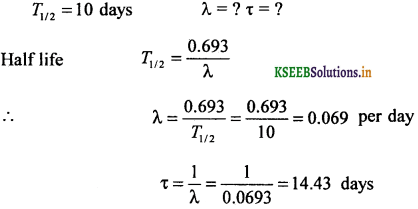Question 3.
The decay constant of a radioactive substance is at the rate of 0.05 per hour. Calculate its half life and mean life.
λ = 0.05 per hour, T = ? τ = ?.
Half life T = $$\frac{0.693}{\lambda}=\frac{0.693}{0.05} \mathrm{hr}$$ = 13.86 hrs.
Mean life τ = $$\frac{1}{\lambda}=\frac{1}{0.05}$$ = 20 hrs.

Question 4.
A radioactive sample of half life T1/2 contains 1000 nuclei. How many will be left after a time t = (T/8) ?Question 5.
A radioactive element has a half life of 20 days. What amount would be left after 80 days, if the original amount was 8 g?
Given, 20 days = 1 half life
80 days = 4 half 1 ives
Initial mass m0 = 8 g
No. of atoms present at any instant is directly proportional to the mass of the sample present at that instant by definition of half life.
No. of atoms left unchanged after n half lives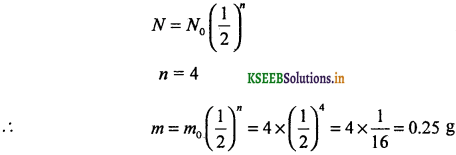Question 6.
A radioactive element has a half life of 20 minutes. Calculate the fraction of element that has decayed in 60 minutes.
Time duration 60 minutes = 3 half lives.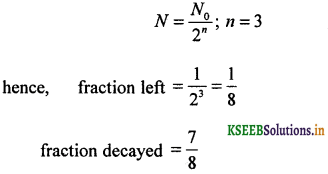Question 7.
Find the activity of 1 gm of radium (ggRa226) whose half life is 1620 years.
No. of atoms in 226 gm is 6.023 × 1023
No. of atoms in 1 gm = $$\frac{6.023 \times 10^{23}}{226}$$ = 2.665 × 1023
Activity ‘A’ = λN; 1 year = 365.25 × 24 × 3600 = 31.5576 × 106 s
λ = $$\frac{0.693}{\mathrm{T}}$$ where T = 1 yearA = 3.612 × 1010 disintegration / sec.

Question 8.
A radioactive sample has a half life of 30 minute. How long will it take for $$\frac{5}{6}$$ th of the sample to disintegrate?
T1/2 = 30 minutes, initial number of atoms = No. the number of atoms which disintegrates in t minute = $$\left(\frac{5}{6}\right)$$N0
∴ The number of atoms left after t minuteQuestion 9.
Calculate the time required for 40% of a radioactive sample to disintegrate. Half life of the sample is 1.6 × 1o1o years.
T1/2 = 1.6 × 1010 years.
Let t be the time in which 40% of the sample disintegrates
∴ after t years 60% of the sample will be left over
i.e., $$\frac{60}{100}$$N0 = N0e-λt
∴ λt = 2.303(log10 100 – log10 60) = 2.303 (0.2219)t = 1.032 × 1010 years.

Question 10.
A certain radioactive sample decays for a time period equal to its mean life. Find
(i) the fraction of the sample that disintegrates and
(ii) the fraction of the sample that remains intact.
Time of decay, t = τ = $$\frac{1}{\lambda}$$
The fraction of sample that remains intact = $$\frac{N}{N_{0}}$$Fraction of the sample that remains intact = 0.368.
Fraction of the sample that disintegrates = 1 – 0.368 = 0.632.

Question 11.
Calculate the specific binding energy of the nucleus of 147N. Given the rest mass of nucleus of 147N= 14.00307 u, the rest mass of proton = 1.00783 u and the rest mass of neutron = 1.00867 u.
Calculation of mass defect:
Δm = Zmp + (A – Z) mn – M
Δm = 7 × 1.00783 + 7 × 1.00867 – 14.00307 = 0.11243 u
Calculation of binding energy:
For 1 u (lamu), binding energy, ΔE = 931.5 MeV
For 0.11243 u(lamu), ΔE = 0.11243 × 931.5MeV
= 104.72854 MeV .
Binding energy per nucleon = $$\frac{B E}{A}$$ =7.4861 MeV/nucIeonQuestion 12.
Determine the mass of 10 kCi sample of 11Na24 whose half life is 20 hours.
T1/2 = 20 hours. A = 10 × (10)3 × 3.7 × 1010 = 3.7 × 1014 / second, m = ?
A = λN
∴ Number of atoms present in the given sample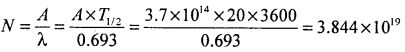Mass of 6.023 × 1026 atoms of Na24 is 24 kg
Mass of 3.844 × 1019 atoms, m = $$\frac{24 \times 3.844 \times 10^{19}}{6.023 \times 10^{26}}$$ = 1.53 × 10-6 kg

Question 13.
Determine the mass of Na22 which has an activity of 5 mCi. Half life of Na22 is 2.6 years. Avogadro number = 6.023 × 1023 atoms. (March 2015)
Given A = 5 × 10-3 × 3.7 × 1010 dis. s-1 = 18.5 × 107 dis.s-1.
Half life period T = 2.6 × 365 × 86400 = 8.2 × 107s.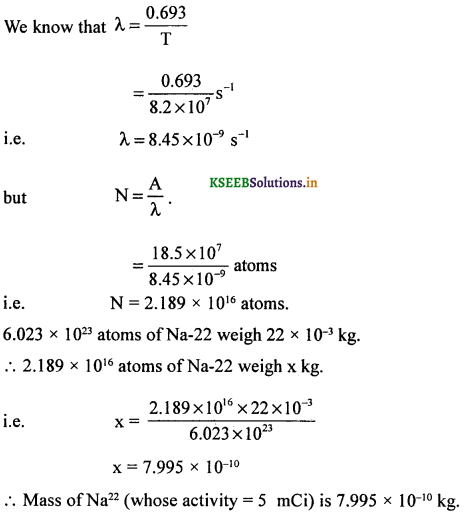Question 14.
A radio active isotope has a half-life of ‘T’ years. How long will it take the activity to reduce to
(a) 3.125%
(b) 1% of the original value.Question 15.
The radio nuclide 11C decays according to 116C → 115 B + e + v. The maximum energy of the emitted positron is 0.960 MeV. Given, the rest mass of C – 11 = 11.011434 u and rest mass of B – 11 = 11.009304 u, calculate ‘Q’ and compare it with the maximum energy of the positron emitted.
The rest mass of the products:positron carries 97.5% of the total energy released.

Question 16.
How long can an electric bulb of 100 W be kept glowing by the fusion of 2.0 kg of deuterium? Take the fusion reaction as 21H + 21H → 42He + 3.27MeV
2 of 21H consists of 6.023 × 1023 atoms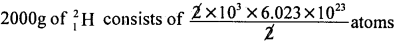i.e., N = 6.023 × 1026 atoms
Number of possible reactions = $$\frac{\mathrm{N}}{2}$$ = 3.012 × 1026
Energy released in the fusion = 3.012 × 1026 × 3.27 MeV
= 9.849 × 1026 MeV
= 9.849 × 1023 × 1.6 × 10-19 × 106 J
= 15.76 × 1013J
Given power of lamp = 100 W
= 100 Js- ‘
i.e., to spend 100 J it takes 1s, for 15.76 × 1013 J of energy to spend, it takes ‘x’ s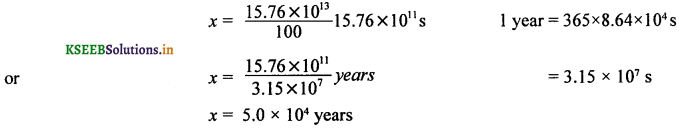Question 17.
Estimate the order of nuclear density.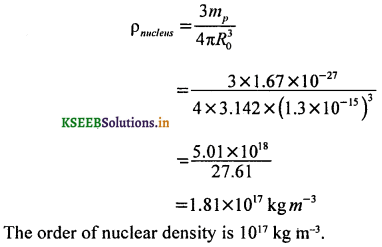Question 18.
A source contains two phosphorus radio nuclides 3315P(T1/2 = 14.3d) and 3215P(T1/2 = 25.3d). Initially, 10% of the decays come from 3315P. How long must one wait until 90% do so?
Initially the source has 90% of 3315P nuclides. After (say) t days, the source has 10% of 3315P nuclides and 90% 3215P nuclides.
∴ Initial number of 3215P nuclides = 9x and 33P = x
Final number of 32P nuclides = y and of 33P = 9y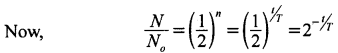i.e., N = N02-1/T
For the 32P isotope, N0 = 9x, N = y; T = 14.3 days
y = 9x(2)-t/143 …… (1)
For the 33P isotope, N0 = x, N = 9y; T = 25.3 days
9y = x(2)-t/25.3 …… (2)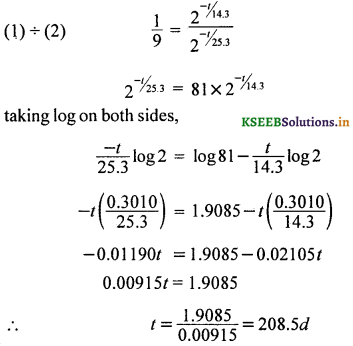Question 19.
Obtain the maximum kinetic energy of β – particle and the radiation frequencies corresponding to γ – decays in the figure given below, (rest mass of Au – 198.968233 u and r.m. of Hg = 198 = 197.966760 u)
Energies of γ – photons γ1, γ2, γ3 are
E1 = (1.088 – 0) MeV = 1.088 MeV = 1.088 × 1.6 × 10-13 = 1.7408 × 10-13 J
E2 = (0.412 – 0) MeV = 0.412 MeV = 0.412 MeV = 0.412 × 1.6 × 10-13 = 0.6592 × 10-13 J
E3 = (1.088 – 0.452)MeV = 0.676 MeV = 0.672 × 1.6 × 10-13 = 1.0816 × 10-13 J
but frequency of radiation v = $$\frac{E}{h}$$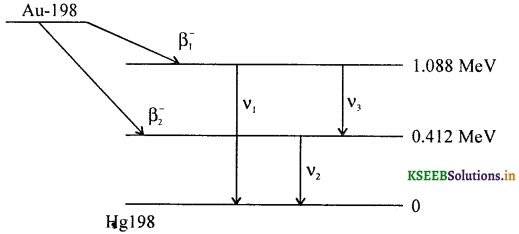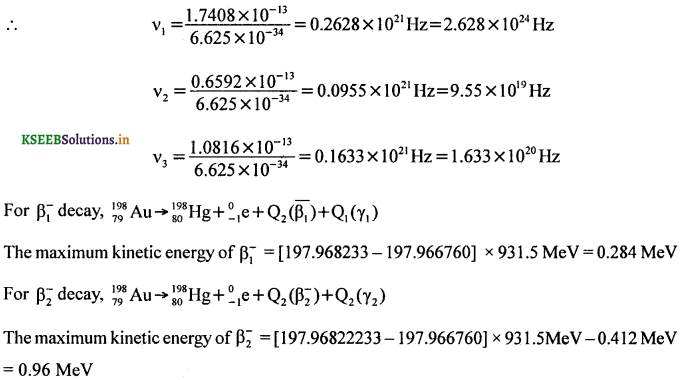Question 20.
Activity of a certain radioactive sample is 25 µCi at the end of 20 days and 6.25 µCi at the end of 40 days. What will be its activity at the end of 30 days? (NCERT)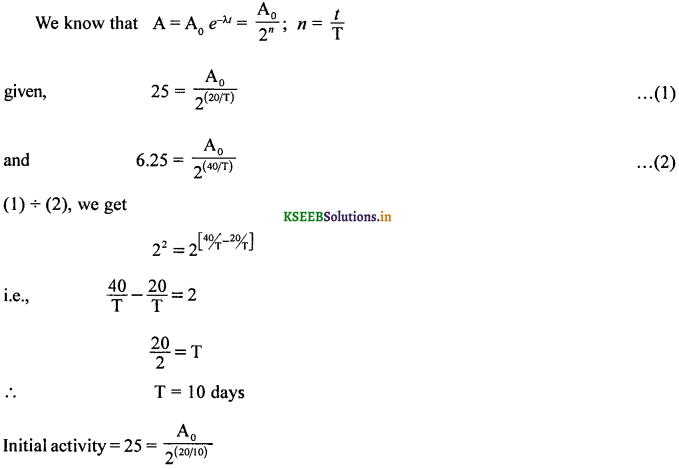Question 21.
Suppose India has a target of producing by 2020AD, 200 GW of electric power, ten percent of which is to be obtained from nuclear power plants. Suppose the efficiency remained at 25%, what amount of fuel may be required per year by 2020? Take the heat energy per fission of 235U to be about 200 MeV.
Nuclear power to be generated = 10% of 200 hw = 20 GW
At 25% efficiency actual power required = $$\frac{100}{25}$$ × 20GW = 80 GW
Total energy required for 1 year production of power = 80 × 109 × 3 65 × 8.64 × 104 J
i.e., E = 2.523 × 1018 J
Energy per fission = 200 MeV
= 200 × 1.6 × 1013 J
= 320.0 × 1013 J
E’ = 3.2 × 10-11 J
Total number of fission atoms required = $$\frac{2.523 \times 10^{18}}{3.2 \times 10^{-11}}$$ = 7.884 × 1028.
But 6.023 × 1023 atoms of Uranium have a mass of 235g.
∴ 7.884 × 1030 atoms of Uranium have a mass of xg.
i.e., x = $$\frac{235 \times 7.884 \times 10^{28}}{6.023 \times 10^{23}}$$
x = 307.6 × 105
Hence, m = 307.6 × 102 kg
= 3.076 × 104 kg
or m = 30.76 tons.Question 22.
Consider the D-T reaction (fusion of deuterium – tritium) 21H + 31H → 42He + n
(a) Calculate the energy released in MeV in this reaction from the data:
m(21H) = 2.014102u; m(42He) = 4.002604u
m(31H) = 3.0016049u;m(n) = 1.00867u

(b) Consider the radius of both deuterium and tritium to be approximately 2.0fm. What is the kinetic energy needed to overcome the coulomb repulsion between the two nuclei? To what temperature must the gas be heated to initiate the reaction? (NCERT)
(a)Mass defect Δm = (2.014102 + 3.016049) – (4.002604 + 1.00867)
= (5.030151 – 5.011274) = 0.018877 U
Energy equivalent is 0.018877 × 931.5 MeV = 17.5839 MeV
= 17.5839 × 1.6 × 1o-13 J
E = 28.134 × 1o-13 J

(b) Potential energy of 21H and 31H during head – on collision is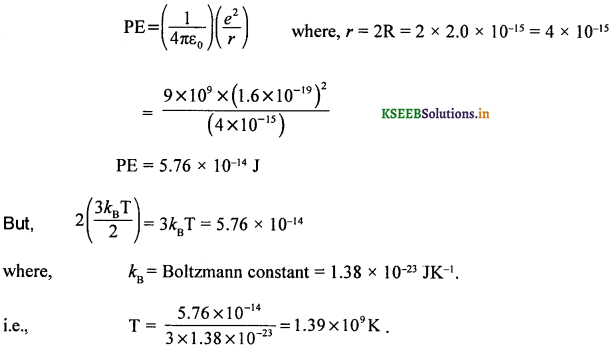Question 23.
Calculate the energy required to separate a neutron (neutron separation energy) from the nucleus 4120Ca. Given r.m. of 4120Ca = 40.962278 U; r.m of 4020Ca = 39.962591 U; r.m. of 1n =1.00867 U.
4120Ca + Q → 4020Ca + 10n
i.e., Q = [39.96259 1 + 1.00867 – 40.962278] × 931.5 MeV = 8.36 MeV
Energy required to separate a neutron = 8.36 MeV

Question 24.
Show that N = $$\frac{N_{0}}{2^{n}}$$ where n = number of half lives n = t/T
Let N0 be the initial a number of atoms be present in the sample. The number of half lives n = t/T where’T’ is the half life period.
During the first half life period,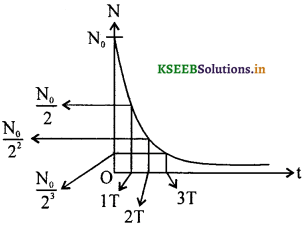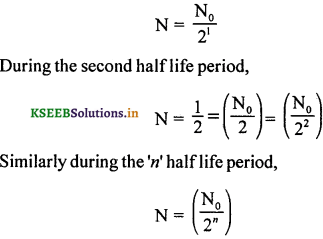Question 25.
From the relation R = RoA1/3, where ‘R0‘ is a constant and ‘A’ is the mass number of a nucleus, show that the nuclear matter density is nearly constant.